# Intersecting Lines

Here we will learn about intersecting lines, including how to find the point of intersection of two straight lines and how to solve simultaneous equations graphically and algebraically.

There are also intersecting lines worksheets based on Edexcel, AQA and OCR exam questions, along with further guidance on where to go next if you’re still stuck.

## What are intersecting lines?

Intersecting lines are when two or more lines cross each other in a plane. There is one common point which lies on both lines which is called the point of intersection.

Two straight intersecting lines create pairs of vertically opposite angles (or vertical angles). One of the important properties of intersecting lines is that each pair of vertically opposite angles is equal.

Step-by-step guide: Vertically opposite angles

In the Cartesian coordinate plane (using x and y, as we do at GCSE), two given lines l_{1} and l_{2} have either one intersection point, or no intersection points.

If a pair of lines do not intersect and have no common point, they are parallel.

Remember that parallel lines have the same gradient.

Perpendicular lines intersect at right angles.

The product of perpendicular gradients is -1.

This idea links coordinate geometry to algebra work on simultaneous equations. If we are given the equations of two intersecting lines, or a graph of the two equations, the point of intersection of two lines is the solution to that pair of linear simultaneous equations.

In three dimensions, it is possible to have two lines which are not parallel but also not in the same plane – these are called skew lines, and will not intersect.

### What are intersecting lines?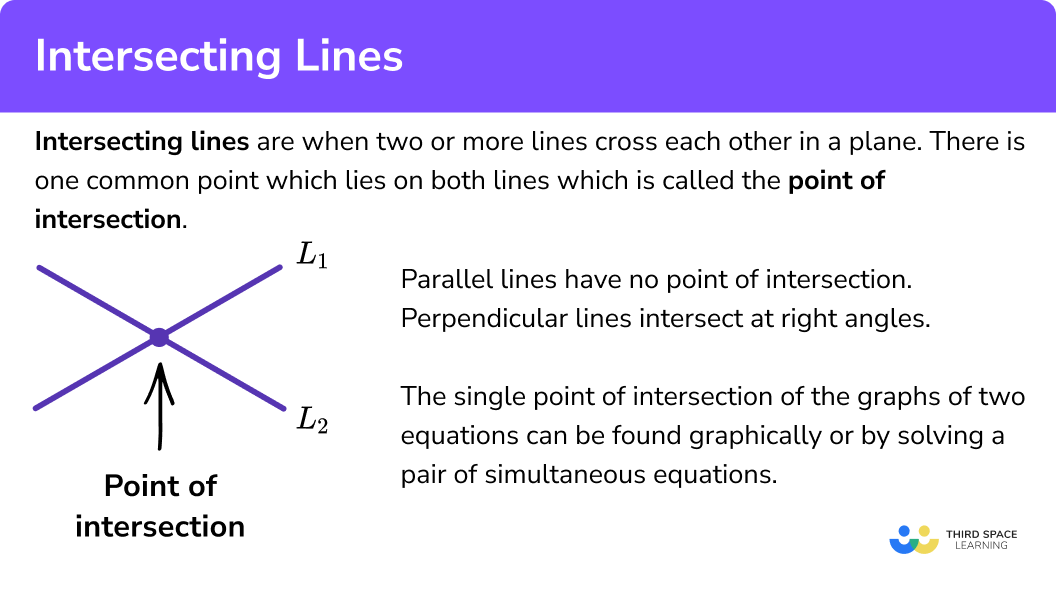## How to use intersecting lines

In order to find the point of intersection using a graph:

1. Plot the graph of the first equation.
2. On the same set of axes, plot the graph of the second equation.
3. Write down the coordinates of the point of intersection.

### Explain how to use intersecting lines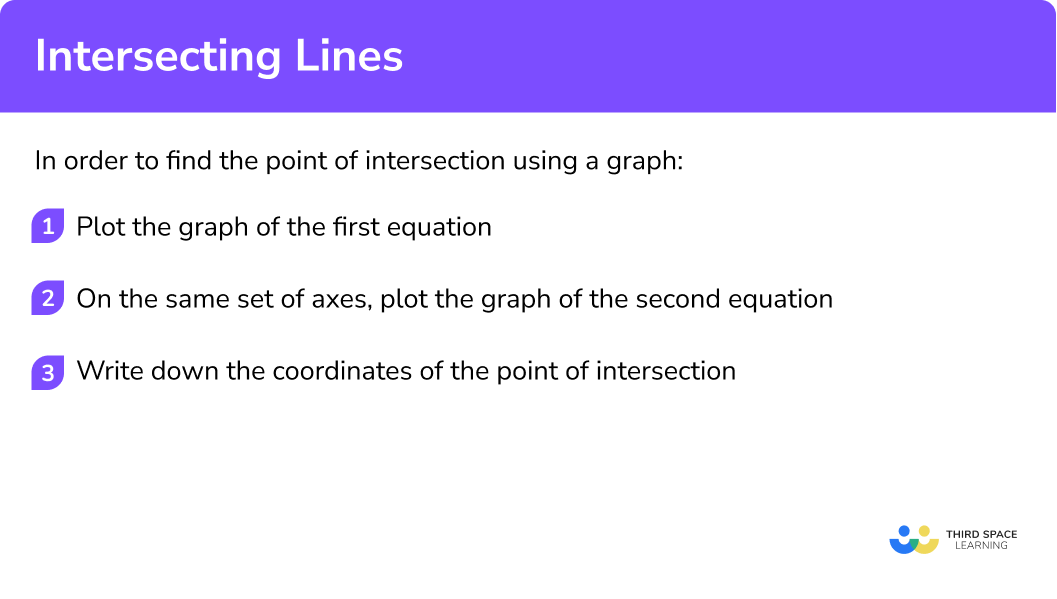### Related lessons on straight line graphs

Intersecting lines is part of our series of lessons to support revision on straight line graphs. You may find it helpful to start with the main straight line graphs lesson for a summary of what to expect, or use the step by step guides below for further detail on individual topics. Other lessons in this series include:

## Point of intersection using a graph examples

### Example 1: finding the point of intersection using a graph

Find the point of intersection of the lines y=x+4 and y=2x-3.

1. Plot the graph of the first equation.

First plot a graph of the equation y=x+4.

Draw a table of values ( 3 or 4 points are sufficient).

x 0 4 8 12
y 4 8 12 16

Step-by-step guide: How to plot a straight line graph

2On the same set of axes, plot the graph of the second equation.

Next plot the graph of the second equation y=2x-3 on the same set of axes.

Draw a table of values ( 3 or 4 points are sufficient).

x 0 4 8 12
y -3 5 13 21

3Write down the coordinates of the point of intersection.

Simply read the coordinates from the graph – the point of intersection is (7,11).

In GCSE Mathematics exams, you may given one of these lines, and asked to plot the other before finding the point of intersection.

You might not always be asked specifically to “find the point of intersection” – the question might say something like “use your graph to solve the pair of simultaneous equations y=x+4 and y=2x-3 ”.

Step-by-step guide: Solving simultaneous equations graphically

### Example 2: finding the point of intersection using a graph

Find the point of intersection of the lines 3x+4y=20 and 2x-2y=4.

First plot a graph of the equation 3x+4y=20.

You may find it easier to rearrange the equation into the form y=-\frac{3}{4}x+5

Draw a table of values ( 3 or 4 points are sufficient).

x 0 4 8
y 5 2 -1

Next plot the graph of the second equation 2x-2y=4 on the same set of axes.

This could be written as y=x-2

Draw a table of values ( 3 or 4 points are sufficient).

x 0 4 8
y -2 2 6

Read the coordinates from the graph – the point of intersection is (4,2).

## Finding the point of intersection algebraically

Sometimes the point of intersection is not easy to read from a graph (for example, if it is a fraction or decimal) and drawing a graph is quite a long process.

If we are working with two straight lines and we have their equations, we can solve them algebraically as a pair of simultaneous equations to find the point of intersection.

Step-by-step guide: Simultaneous equations

## How to find the point of intersection algebraically

In order to find the point of intersection algebraically, we follow the steps for solving simultaneous linear equations:

1. Eliminate one variable.
2. Find the value of the other variable.
3. Find the value of the remaining variable via substitution.

### Explain how to find the point of intersection algebraically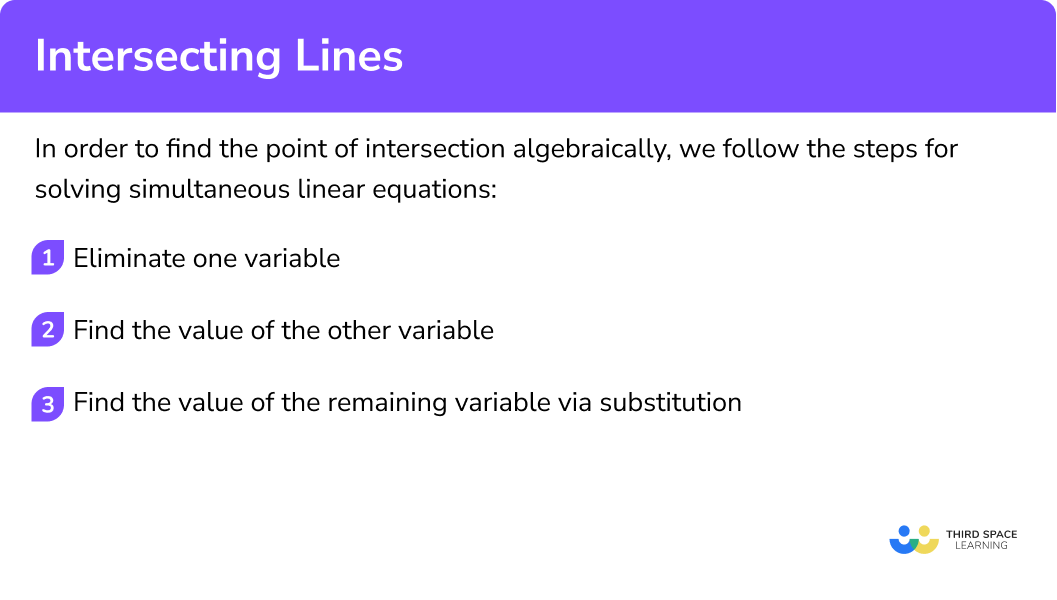## Point of intersection using simultaneous equations examples

### Example 3: finding the point of intersection algebraically

Find the point of intersection of the lines 3x+4y=20 and 2x-2y=4.

Note that this is the same pair of equations we considered in example 2 above – here we will look at the algebraic method, which results in the same answer.

\begin{aligned} &(1) \ 3x+4y=20 \\ &(2) \ 2x-2y=8 \end{aligned}

Multiply equation (2) by 2 to get,

\begin{aligned} &(1) \ 3x+4y=20 \\ &(2) \ 4x-4y=8 \end{aligned}

Then add the two equations together to eliminate y.

7x=28

\begin{aligned} &7x=28\\\\ &x=4 \end{aligned}

We now know that x=4, so we can find the value of y by substituting this value of x into either of the two original equations.

\begin{aligned} 3 x+4 y&=20 \\\\ 3(4)+4 y&=20 \\\\ 12+4 y&=20 \\\\ 4 y&=8 \\\\ y&=2 \end{aligned}

So the point of intersection is (4, 2).

### Example 4: Finding the point of intersection algebraically

Find the point of intersection of the lines 2x+3y=20 and y=x-5.

Here we can substitute the expression y=x-5 into 2x+3y=20.

\begin{aligned} 2 x+3 y&=20 \\\\ 2 x+3(x-5)&=20 \\\\ 2 x+3 x-15&=20 \\\\ 5 x-15&=20 \end{aligned}

\begin{aligned} 5 x-15 &=20 \\\\ 5 x &=35 \\\\ x &=7 \end{aligned}

We now know that x=7, so we can find the value of y by substituting this value of x into either of the two original equations.

\begin{aligned} 2 x+3 y &=20 \\\\ 2(7)+3 y &=20 \\\\ 14+3 y &=20 \\\\ 3 y &=6 \\\\ y &=2 \end{aligned}

So the point of intersection is (7, 2).

## Showing that intersecting lines are parallel or perpendicular

Two lines are parallel if they have the same gradient.

Two lines are perpendicular if the product of their gradients is -1.

By considering the gradients of the two lines, we can decide whether they are parallel, perpendicular or neither.

Step-by-step guide: Parallel and perpendicular lines

## How to show that lines are parallel or perpendicular

In order to decide whether two lines are parallel, perpendicular or neither:

1. Rearrange both equations to the form y=mx+c .
2. Compare the gradients to decide if they are parallel, perpendicular or neither.

### Explain how to show that lines are parallel or perpendicular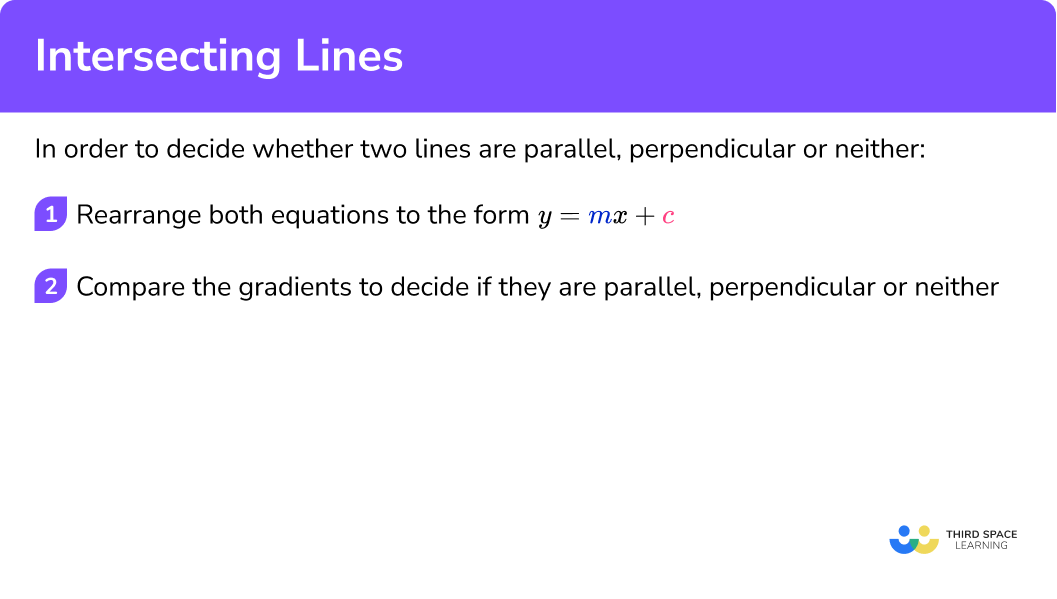### Example 5: a pair of parallel lines

Show that the lines y=3x+5 and 6x-2y=24 are parallel and do not intersect.

The first equation is already in the correct form.

Rearrange the second equation.

\begin{aligned} 6 x-2 y &=24 \\\\ 6 x &=2 y+24 \\\\ 6 x-24 &=2 y \\\\ 3 x-12 &=y \end{aligned}

The equations of the lines are,

\begin{aligned} &y=3x+5 \\\\ &y=3x-12 \end{aligned}

The gradient of each equation is 3, hence the lines are parallel. Since they are parallel they do not intersect.

### Example 6: a pair of perpendicular lines

Show that the lines 6x+2y=30 and 3y-x=24 are perpendicular and intersect at right angles.

Rearrange the first equation.

\begin{aligned} 6 x+2 y&=30 \\\\ 2 y&=30-6 x \\\\ y&=15-3 x \end{aligned}

Rearrange the second equation.

\begin{aligned} 3 y-x &=24 \\\\ 3 y &=24+x \\\\ y &=8+\frac{1}{3} x \end{aligned}

The equations of the lines are,

\begin{aligned} &y=15-3 x \\\\ &y=8+\frac{1}{3} x \end{aligned}

Multiplying the gradients together to find their product,

-3 \times \frac{1}{3}=\frac{-3}{3}=-1.

The product of the gradients is -1, so the lines are perpendicular and intersect at 90 degrees.

### Common misconceptions

• Concluding that two lines do not intersect because the point of intersection is not visible in the drawn range of the graph

For example, the lines y=2x+4 and y=4x-5 do actually intersect at the point (4.5, 13), although this is not visible on the graph drawn below.

• Not rearranging to the form y=mx+c when comparing gradients

For example, the equations y=2x+7 and 2y+2x=4 are not parallel, even though the coefficient of x is 2 in each case. Here you would need to rearrange the second equation to y=2-x. We can now see that the gradient is -1 and the lines are therefore not parallel.

### Practice intersecting lines questions

1. Find the point of intersection of the lines graphically,

\begin{aligned} &2x+2y=10\\ &y=2x+2 \end{aligned}

(2, 3)(1, 4)(4, 1)(3, 8)Draw a table of values for each equation and plot as below.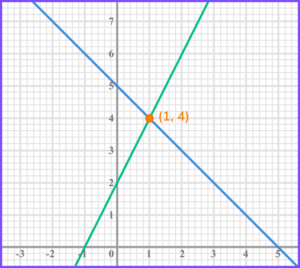Read the point of intersection from the graph (1,4).

2. Find the point of intersection of the lines graphically,

\begin{aligned} &3x+5y=40\\ &y=3x-10 \end{aligned}

(2, -4)(10, 2)(6, 8)(5, 5)Draw a table of values for each equation and plot as below.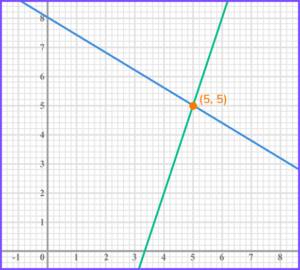Read the point of intersection from the graph (5,5).

3. Find the point of intersection of the lines algebraically,

\begin{aligned} &3x+3y=30\\ &2x-3y=10 \end{aligned}

(8, 2)(20, 10)(5, 5)(2, 8)Add the two equations together to eliminate y and get 5x=40. Solve to get x=8. Then substitute x=8 into one of the original equations to get y=2.

4. Find the point of intersection of the lines algebraically,

\begin{aligned} &5x+2y=50\\ &y=2x-2 \end{aligned}

(8, 5)(3, 4)(6, 10)(3, 10)Substitute y=2x-2 into 5x+2y=50 to get 5x+2(2x-2)=50. Expand the brackets and solve to get x=6. Finally substitute into one of the original equations to get y=10.

5. Choose the correct option for this pair of lines,

\begin{aligned} &y=4x-10 \\ &12x-3y=24 \end{aligned}

Parallel, no intersectionPerpendicular, the points intersect at (4, 6)Neither, the points intersect at (4, 6)Neither, the points intersect at (2, 0)Rearranging 12x-3y=24 to 12x-24=3y and then dividing by 3 gives the equation y=4x-8. Both lines have the same gradient (4), so they are parallel and do not intersect.

6. Choose the correct option for this pair of lines,

\begin{aligned} &y=5x-4 \\ &2y=3x+6 \end{aligned}

Parallel, no intersectionPerpendicular, the points intersect at (2, 6)Neither, the points intersect at (2, 6)Neither, the points intersect at (4, 5)Multiply the first equation by 2 to get 2y=10x-8, then set this equal to the second equation to get 10x-8=3x+6.

Solve the equation to get x=2, and substitute into one of the original equations to get y=6.

Finally, check the gradients. Dividing the equation 2y=3x+6 by 2 gives y=\frac{3}{2} x+3. So the two gradients are 5 and \frac{3}{2}. These do not have a product of -1, so the lines are not perpendicular.

### Intersecting lines GCSE questions

1. Here is the graph of the equation 3x+4y=48.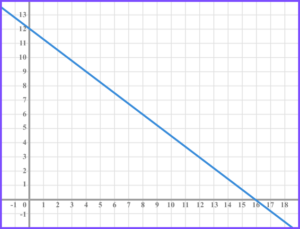(a) On the same set of axes, draw the graph of y=2x+1.

(b) Hence or otherwise, solve the simultaneous equations,

\begin{aligned} &3x+4y=48\\ &y=2x+1 \end{aligned}

(3 marks)

(a)

Straight line with gradient 2 or y -intercept 1 .

(1)

Fully correct line.

(1)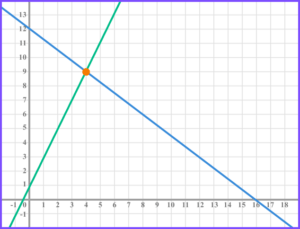(b)

x=4, \ y=9

(1)

2. Find the point of intersection of the lines,

\begin{aligned} &y=2x+5\\ &y=3x-2 \end{aligned}

(3 marks)

2x+5=3x-2

(1)

x = 7

(1)

y=19 so coordinate is (7,19) .

(1)

3. (a) Show that the lines l_{1}: y+4 x+14=0 and  l_{2}: y=\frac{1}{4} x+20 intersect at the point P \: (-8, 18).

(b) Show that the two lines intersect at right angles.

(6 marks)

(a)

Substitute -8 into y+4x+14=0 to get,

\begin{aligned} y+4(-8)+14 &=0 \\\\ y-32+14 &=0 \\\\ y &=18 \\\\ \end{aligned}

So P lies on l_{1} .

(1)

Substitute -8 into y=\frac{1}{4} x+20 to get,

\begin{aligned} &y=\frac{1}{4}(-8)+20 \\\\ &y=-2+20 \\\\ &y=18 \\\\ \end{aligned}

So P lies on l_{2} .

(1)

P lies on l_{1} and l_{2}, so the two lines must intersect at P.

(1)

Alternative solution:

Eliminate one variable i.e.

\frac{1}{4} x+2-=-14-4 x

(1)

Solve to get x=-8 .

(1)

Substitute x=-8 into one of the equations e.g.

y=\frac{1}{4}(-8)+20=18

(1)

(b)

Rearrange to find gradient of l_{1},

\begin{aligned} y+4 x+14 &=0 \\ y &=-4 x-14 \end{aligned}

(1)

(1)

\frac{1}{4}\times -4=-1 hence the lines are perpendicular and intersect at right angles.

(1)

## Learning checklist

You have now learned how to:

• Find the point of intersection of two lines graphically
• Find the point of intersection algebraically
• Show that two lines are parallel or perpendicular

## Still stuck?

Prepare your KS4 students for maths GCSEs success with Third Space Learning. Weekly online one to one GCSE maths revision lessons delivered by expert maths tutors.

Find out more about our GCSE maths tuition programme.